Daily Practice Problems
Class 11 Maths
Sequences and SeriesQuestion 1:

In an AP, if mth term is n and the nth term is m, where m ≠ n, find the pth term.

Question 2:

A man saved Rs. 66000 in 20 years. In each succeeding year after the first year, he saved Rs. 200 more than what he saved in the previous year. How much did he save in the first year?

Question 3:

A carpenter was hired to build 192 window frames. The first day he made five frames and each day, thereafter he made two more frames than he made the day before. How many days did it take him to finish the job?

Question 4:

If 2x, x + 8, 3x + 1 are in A.P, then what is the value of x?

Question 5:

Find the sum of the series (33 – 23) + (53 – 43) + (73 – 63) + … to n terms.

Question 6:

If 9 times the 9th term of an A.P. is equal to 13 times the 13th term, then find the 22nd term of the A.P.

Question 7:

Write the first five terms of the sequences whose nth term is an = n *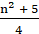Question 8:

If Tn denotes the nth term of the series 2 + 3 + 6 + 11 + 18 + …, then T50 is ____.

Question 9:

In an A.P. if pth term is 1qand qth term is1p, prove that the sum of first pq terms is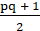, where p ≠ q. where p ≠ q.

Question 10:

If x, 2y and 3z are in A.P. where the distinct numbers x, y and z are in G.P., then find the common ratio of the G.P.

Question 11:

The sums of n terms of two arithmetic progressions are in the ratio 5n + 4 : 9n + 6. Find the ratio of their 18th terms.

Question 12:

Find the sum to n terms of the sequence: 3, 33, 333, 3333, ......

Question 13:

In a G.P., the 3rd term is 24 and the 6th term is 192. Find the 10th term.

Question 14:

If a, b, c are in GP then log an, log bn, log cn are in _____.

Question 15:

A person has 2 parents, 4 grandparents, 8 great grandparents, and so on. Find the number of his ancestors during the ten generations preceding his own.

Question 16:

The sum of n terms of the series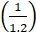+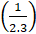+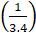+ …… is _____.

Question 17:

The first term of a GP is 1. The sum of the third term and fifth term is 90. The common ratio of GP is ______.

Question 18:

Find the value of n so that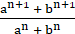may be  may be the geometric mean between a and b.

Question 19:

Find the sum to n terms of the series 3 * 8 + 6 * 11 + 9 * 14 +........

Question 20:

Find the sum to n terms of the series whose nth term is n(n + 3).

**********